Next: Exponential Growth and Decay Up: Applications of the Natural Previous: Applications of the Natural

## Background

In order to enter the exponential function,, in Maple, you must use the exp command. The syntax of this command is similar to that of sin and cos. For example, here we enter the function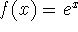and then evaluate at x = 2.

```  > f:=exp(x);
```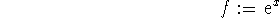```  > evalf(subs(x=2,f));
```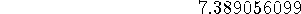The natural log function, ln, is also used in this manner.

```  > g:=ln(x);
```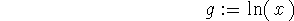```  > evalf(subs(x=2,g));
```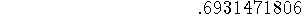Plotting the two functionsandon the same coordinate system gives an idea of the symmetry around the line y=x. Make sure that you can identify which curve isand which curve is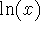.

```  > plot(f,g,x,x=-5..5,y=-5..5);
```

Looking at the graph of the two functions, you can get a pretty good idea of what happens to f(x) as x approaches negative infinity; however,in order to be sure, you must take a limit.

```  > limit(f,x=-infinity);
```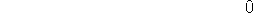Roxanne Tisch
Tue Sep 24 13:59:38 EDT 1996